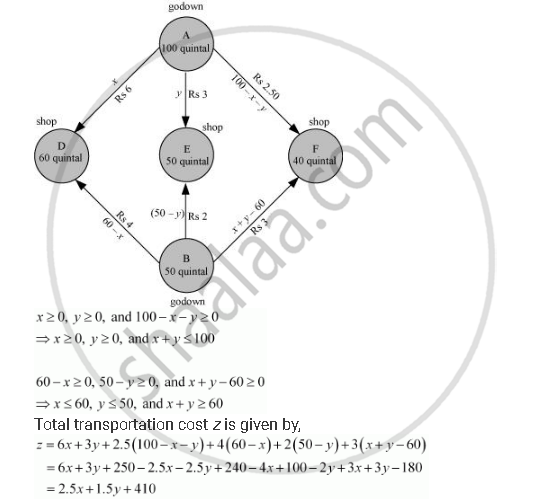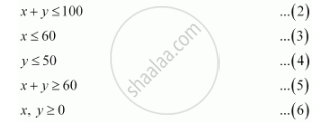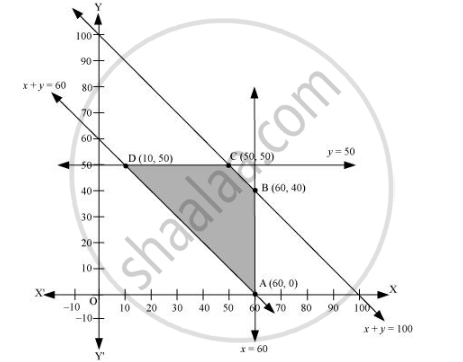Share

# Two Godowns a and B Have Grain Capacity of 100 Quintals and 50 Quintals Respectively. They Supply to 3 Ration Shops, D, E and F Whose Requirements Are 60, 50 and 40 Quintals Respectively How Should the Supplies Be Transported in Order that the Transportation Cost is Minimum? What is the Minimum Cost? - CBSE (Commerce) Class 12 - Mathematics

ConceptDifferent Types of Linear Programming Problems

#### Question

Two godowns A and B have grain capacity of 100 quintals and 50 quintals respectively. They supply to 3 ration shops, D, E and F whose requirements are 60, 50 and 40 quintals respectively. The cost of transportation per quintal from the godowns to the shops are given in the following table:

 Transportation cost per quintal (in Rs) From/To A B D 6 4 E 3 2 F 2.50 3

How should the supplies be transported in order that the transportation cost is minimum? What is the minimum cost?

#### Solution

Let godown A supply x and y quintals of grain to the shops D and E respectively. Then, (100 − x − y) will be supplied to shop F.

The requirement at shop D is 60 quintals since x quintals are transported from godown A. Therefore, the remaining (60 −x) quintals will be transported from godown B.

Similarly, (50 − y) quintals and 40 − (100 − x − y) = (x + y − 60) quintals will be transported from godown B to shop E and F respectively.

The given problem can be represented diagrammatically as follows.The given problem can be formulated as

Minimize z = 2.5x + 1.5y + 410 … (1)

subject to the constraints,The feasible region determined by the system of constraints is as follows.The corner points are A (60, 0), B (60, 40), C (50, 50), and D (10, 50).

The values of z at these corner points are as follows.

 Corner point z = 2.5x + 1.5y + 41 A (60, 0) 560 B (60, 40) 620 C (50, 50) 610 D (10, 50) 510 → Minimum

The minimum value of z is 510 at (10, 50).

Thus, the amount of grain transported from A to D, E, and F is 10 quintals, 50 quintals, and 40 quintals respectively and from B to D, E, and F is 50 quintals, 0 quintals, and 0 quintals respectively.

The minimum cost is Rs 510.

Is there an error in this question or solution?

#### Video TutorialsVIEW ALL 

Solution Two Godowns a and B Have Grain Capacity of 100 Quintals and 50 Quintals Respectively. They Supply to 3 Ration Shops, D, E and F Whose Requirements Are 60, 50 and 40 Quintals Respectively How Should the Supplies Be Transported in Order that the Transportation Cost is Minimum? What is the Minimum Cost? Concept: Different Types of Linear Programming Problems.
S